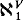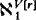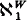Hostname: page-component-797576ffbb-gvrqt Total loading time: 0 Render date: 2023-12-08T09:52:18.824Z Has data issue: false Feature Flags: { "corePageComponentGetUserInfoFromSharedSession": true, "coreDisableEcommerce": false, "useRatesEcommerce": true } hasContentIssue false

# Forcing the failure of CH by adding a real

Published online by Cambridge University Press:  12 March 2014

## Extract

We prove several independence results relevant to an old question in the folklore of set theory. These results complement those in [Sh, Chapter XIII, §4]. The question is the following. Suppose V ⊨ “ZFC + CH” and r is a real not in V. Must V[r] ⊨ CH? To avoid trivialities assume=.

We answer this question negatively. Specifically we find pairs of models (W, V) such that W ⊨ ZFC + CH, V = W[r], r a real,=and V ⊨ ¬CH. Actually we find a spectrum of such pairs using ZFC up to “ZFC + there exist measurable cardinals”. Basically the nicer the pair is as a solution, the more we need to assume in order to construct it.

The relevant results in [Sh, Chapter XIII] state that if a pair (of inner models) (W, V) satisfies (1) and (2) then there is an inaccessible cardinal in L; if in addition V ⊨ 20 > ℵ2 then 0# exists; and finally if (W, V) satisfies (1), (2) and (3) with V ⊨ 20 > ℵω, then there is an inner model with a measurable cardinal.

Definition 1. For a pair (W, V) we shall consider the following conditions:

(1) V = W[r], r a real,=, W ⊨ ZFC + CH but CH fails in V.

(2) W ⊨ GCH.

(3) W and V have the same cardinals.

Type
Research Article
Information
The Journal of Symbolic Logic , December 1984 , pp. 1185 - 1189